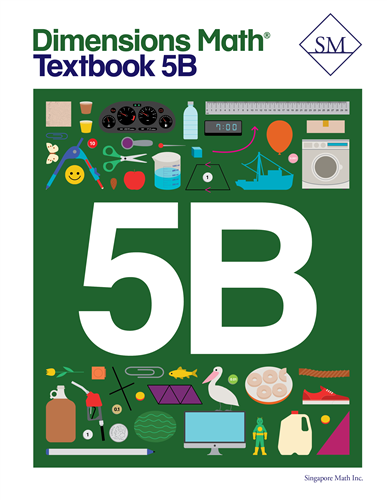# Dimensions Math Textbook 5B

\$ 12.00

Dimensions Math® Textbook 5B

Chapter 9: Decimals

Lesson 1: Thousandths
Lesson 2: Place Value to Thousandths
Lesson 3: Comparing Decimals
Lesson 4: Rounding Decimals
Lesson 5: Practice A
Lesson 6: Multiply Decimals by 10, 100, and 1,000
Lesson 7: Divide Decimals by 10, 100, and 1,000
Lesson 8: Conversion of Measures
Lesson 9: Mental Calculation
Lesson 10: Practice B

Chapter 10: The Four Operations of Decimals

Lesson 1: Adding Decimals to Thousandths
Lesson 2: Subtracting Decimals
Lesson 3: Multiplying by 0.1 or 0.01
Lesson 4: Multiplying by a Decimal
Lesson 5: Practice A
Lesson 6: Dividing by a Whole Number — Part 1
Lesson 7: Dividing by a Whole Number — Part 2
Lesson 8: Dividing by a Whole Number by 0.1 and 0.01
Lesson 9: Dividing a Whole Number by a Decimal
Lesson 10: Practice B

Chapter 11: Geometry

Lesson 1: Measuring Angles
Lesson 2: Angles and Lines
Lesson 3: Classifying Triangles
Lesson 4: The Sum of the Angles in a Triangle
Lesson 5: The Exterior Angle of a Triangle
Lesson 7: Angles of Quadrilaterals — Part 1
Lesson 8: Angles of Quadrilaterals — Part 2
Lesson 9: Drawing Triangles and Quadrilaterals
Lesson 10: Practice

Chapter 12: Data Analysis and Graphs

Lesson 1: Average — Part 1
Lesson 2: Average — Part 2
Lesson 3: Line Plots
Lesson 4: Coordinate Graphs
Lesson 5: Straight Line Graphs
Lesson 6: Practice
Review 3

Chapter 13: Ratio

Lesson 1: Finding the Ratio
Lesson 2: Equivalent Ratios
Lesson 3: Finding a Quantity
Lesson 4: Comparing Three Quantities
Lesson 5: Word Problems
Lesson 6: Practice

Chapter 14: Rate

Lesson 1: Finding the Rate
Lesson 2: Rate Problems — Part 1
Lesson 3: Rate Problems — Part 2
Lesson 4: Word Problems
Lesson 5: Practice

Chapter 15: Percentage

Lesson 1: Meaning of Percentage
Lesson 2: Expressing Percentages as Fractions
Lesson 3: Percentages and Decimals
Lesson 4: Expressing Fractions as Percentages
Lesson 5: Practice A
Lesson 6: Percentage of a Quantity
Lesson 7: Word Problems
Lesson 8: Practice B
Review 4
Review 5

Dimensions Math® PreK-5 series features the progression, rigor, and pacing that define Singapore math. Throughout the series, five characters offer students suggestions on how to think about problems. They remind students of strategies they’ve learned and point out important information that encourages them to come up with their own solutions.

Textbook lessons begin with a task that allows students to apply their previous knowledge and learn through discussion. Once students have mastered a concept with the use of concrete and pictorial aids, they are ready to take on more abstract mathematical problem sets. They reach fluency by collecting various strategies along the way and applying them to new problems. Word problems give students a sense of math in real-world contexts.

Workbooks offer independent practice that follows a careful progression of exercise variation. Each textbook lesson includes a corresponding workbook exercise that starts with pictorial representation and progresses to more challenging abstract problems. Workbooks for PreK-2 are perforated.

Teacher’s Guides include lesson plans, mathematical background, games, helpful suggestions, and comprehensive resources for daily lessons. Lessons are laid out clearly and activities are designed for the whole class, small groups, and extension.

Textbooks and Workbooks do not include answer keys. Answers are in Teacher's Guides.
Textbook:
ISBN  9781947226135